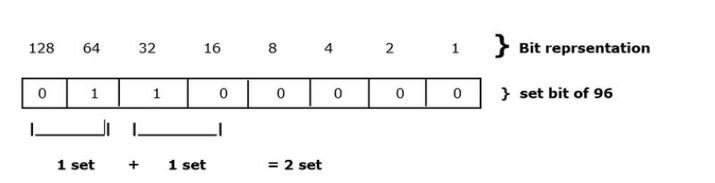# Count of element in an array whose set bits are in a multiple of K

The set bit is a binary representation form of 0 and 1. This digit 1 is called a set bit in reference to the computer. Let’s take an example to understand the setbit calculation −

Let’s take an example to understand the setbit calculation −

The set bit calculation of integer 96 isLet’s say we want to set the bit to sum of 96. So as shown in the above representation, we will set bit 1 to those array elements whose total will be 96. This way we will form 2 sets of bit. Therefore, if we take the K value as 2 then the set bit of 96 is a multiple of it.

In this program, we are going to solve the array element count whose set bits are in a multiple of K.

## Algorithm

• We will start the program with a header file named ‘bits/stdc++.h’ which include all the standard template library of C++.

• We are creating a function definition named ‘find_bitcount’ which accepts three parameters namely arr, n, and k, and define the following −

arr[] − To get the array input from the main function of the array.

n − The length of an array

k − To check the divisibility against a set bit count.

This will calculate the total set bit count of an array element.

• Then we are storing ‘0’ to ‘ans’ variable that will keep track of the count of numbers that meet the condition.

• We are starting the for loop to iterate each element and storing the array element i.e. ‘arr[i]’ to ‘x’ variable which will later meet the condition in while loop to check the condition for total set bits count. This way function initializes ‘x’ to array element values.

• Then initialize the variable ‘setBitsCount’ to ‘0’ which will keep track of the set-bits count of the current array element.

• Next, we create a while loop to check whether x (array element stored in the x) is greater than 0 and do the following −

• setBitsCount += x & 1 − The bitwise & operator with 1 is used in the loop to determine whether the least significant bit of x is 1.

• x = x >> 1 − The set-bits count is increased by 1 if the outcome is 1. The >> operator, which eliminates the least significant bit, is then used in the loop to shift x to the right by 1 bit.

• Now using the if-statement we check if ‘setBitsCount’ is divisible by ‘k’ using the ‘%’ operator and its equivalent to ‘0’ then the current array element meets the condition and increments the variable ‘ans’ by ‘1’.

• After processing all the above conditions, the function returns the value of ‘ans’ which will define the total set bit count of an array element.

• Moving ahead to start the main function and declare all the array elements. Then we are initializing variable ‘n’to find the size of an array and initialize the variable ‘K’ to ‘2’ which will check whether the array element is a multiple of K or not.

• Finally, in the printing statement we are calling the function definition named ‘find_bitcount()’ and get the result.

## Example

In this program, we are going to implement the count of an array element whose set bits are in a multiple of K.

#include <bits/stdc++.h>
#include <bits/stdc++.h>
using namespace std;

// Function to find the count of numbers
int find_bitcount(int arr[], int n, int k) {
int ans = 0;
for (int i = 0; i < n; i++) {
int x = arr[i];
int setBitsCount = 0;

// Calculate the set-bits count of the element x
while (x > 0) {
setBitsCount += x & 1;
x = x >> 1;
}

// Check if the setbits count
// is divisible by K
if (setBitsCount % k == 0)
ans++;
}
return ans;
}
int main() {
int arr[] = { 6, 845, 4, 168, 7896 };
int n = sizeof(arr) / sizeof(arr);
int K = 2;
cout << "There are "<<find_bitcount(arr, n, K)<<" array element whose setbits are in a multiple of K";
return 0;
}


## Output

There are 3 array element whose setbits are in a multiple of K


## Conclusion

We explored the concept of an array element count whose set bits are in a multiple of K. In this program, by defining the function it calculates the total count of set bits array element. Then we observe how set bit counts are shifted by the >> operator and using conditional statement we check how many array elements are passed for set bit count. In the end, we simply print the result.

Updated on: 10-May-2023

164 Views